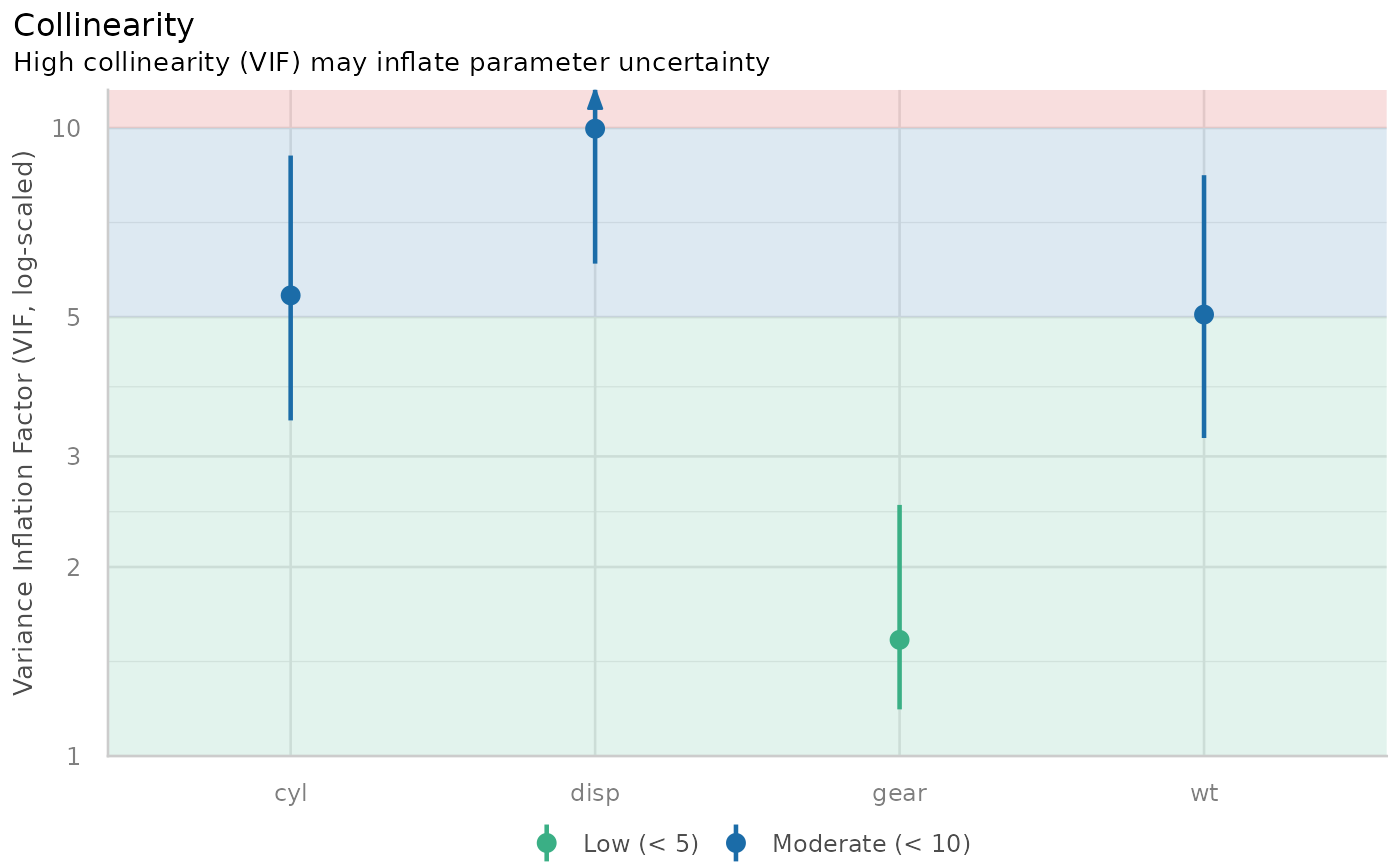check_collinearity() checks regression models for multicollinearity by calculating the variance inflation factor (VIF). multicollinearity() is an alias for check_collinearity(). (When printed, VIF are also translated to Tolerance values, where tolerance = 1/vif.)

check_collinearity(x, ...)

multicollinearity(x, ...)

# S3 method for default
check_collinearity(x, verbose = TRUE, ...)

# S3 method for glmmTMB
check_collinearity(
x,
component = c("all", "conditional", "count", "zi", "zero_inflated"),
verbose = TRUE,
...
)

## Arguments

x A model object (that should at least respond to vcov(), and if possible, also to model.matrix() - however, it also should work without model.matrix()). Currently not used. Toggle off warnings or messages. For models with zero-inflation component, multicollinearity can be checked for the conditional model (count component, component = "conditional" or component = "count"), zero-inflation component (component = "zero_inflated" or component = "zi") or both components (component = "all"). Following model-classes are currently supported: hurdle, zeroinfl, zerocount, MixMod and glmmTMB.

## Value

A data frame with three columns: The name of the model term, the variance inflation factor and the factor by which the standard error is increased due to possible correlation with other terms.

## Details

### Multicollinearity

Multicollinearity should not be confused with a raw strong correlation between predictors. What matters is the association between one or more predictor variables, conditional on the other variables in the model. In a nutshell, multicollinearity means that once you know the effect of one predictor, the value of knowing the other predictor is rather low. Thus, one of the predictors doesn't help much in terms of better understanding the model or predicting the outcome. As a consequence, if multicollinearity is a problem, the model seems to suggest that the predictors in question don't seems to be reliably associated with the outcome (low estimates, high standard errors), although these predictors actually are strongly associated with the outcome, i.e. indeed might have strong effect (McElreath 2020, chapter 6.1).

Multicollinearity might arise when a third, unobserved variable has a causal effect on each of the two predictors that are associated with the outcome. In such cases, the actual relationship that matters would be the association between the unobserved variable and the outcome.

Remember: “Pairwise correlations are not the problem. It is the conditional associations - not correlations - that matter.” (McElreath 2020, p. 169)

### Interpretation of the Variance Inflation Factor

The variance inflation factor is a measure to analyze the magnitude of multicollinearity of model terms. A VIF less than 5 indicates a low correlation of that predictor with other predictors. A value between 5 and 10 indicates a moderate correlation, while VIF values larger than 10 are a sign for high, not tolerable correlation of model predictors (James et al. 2013). The Increased SE column in the output indicates how much larger the standard error is due to the association with other predictors conditional on the remaining variables in the model.

### Multicollinearity and Interaction Terms

If interaction terms are included in a model, high VIF values are expected. This portion of multicollinearity among the component terms of an interaction is also called "inessential ill-conditioning", which leads to inflated VIF values that are typically seen for models with interaction terms (Francoeur 2013).

## Note

There is also a plot()-method implemented in the see-package.

• Francoeur, R. B. (2013). Could Sequential Residual Centering Resolve Low Sensitivity in Moderated Regression? Simulations and Cancer Symptom Clusters. Open Journal of Statistics, 03(06), 24–44.

• James, G., Witten, D., Hastie, T., & Tibshirani, R. (eds.). (2013). An introduction to statistical learning: with applications in R. New York: Springer.

• McElreath, R. (2020). Statistical rethinking: A Bayesian course with examples in R and Stan. 2nd edition. Chapman and Hall/CRC.

• Vanhove, J. (2019). Collinearity isn't a disease that needs curing. webpage

## Examples

m <- lm(mpg ~ wt + cyl + gear + disp, data = mtcars)
check_collinearity(m)
#> # Check for Multicollinearity
#>
#> Low Correlation
#>
#>  Term  VIF Increased SE Tolerance
#>  gear 1.53         1.24      0.65
#>
#> Moderate Correlation
#>
#>  Term  VIF Increased SE Tolerance
#>    wt 5.05         2.25      0.20
#>   cyl 5.41         2.33      0.18
#>  disp 9.97         3.16      0.10

# plot results
if (require("see")) {
x <- check_collinearity(m)
plot(x)
}